### Home > A2C > Chapter 12 > Lesson 12.3.1 > Problem12-132

12-132.
1. Find the exact value for each of the following trig expressions: Homework Help ✎

1.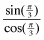2.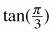3. Explain the relationship between the answers from part (a) and (b) above.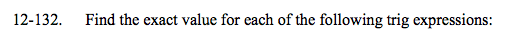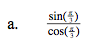Use the unit circle to substitute values for sine and cosine and simplify.

$\sqrt{3}$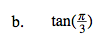$\sqrt{3}$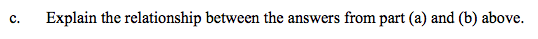What do you know about the relationship between tanθ, sinθ, and cosθ?